UPSC  >  NCERT Summary: Summary of Chemistry- 1

# NCERT Summary: Summary of Chemistry- 1 | Science & Technology for UPSC CSE

 1 Crore+ students have signed up on EduRev. Have you?

Acid, Base and Salts

1. Acid

• The word ‘acid’ is derived from a Latin word, which means “sour”. The sour taste of most of the fruits and vegetables is due to various types of acids present in them. The digestive fluids of most animals and humans also contain acids.
• An acid is a compound, which on dissolving in water yields hydronium ions (H3O+) as the only positive ions. The characteristic property of acid is due to the presence of these hydronium ions.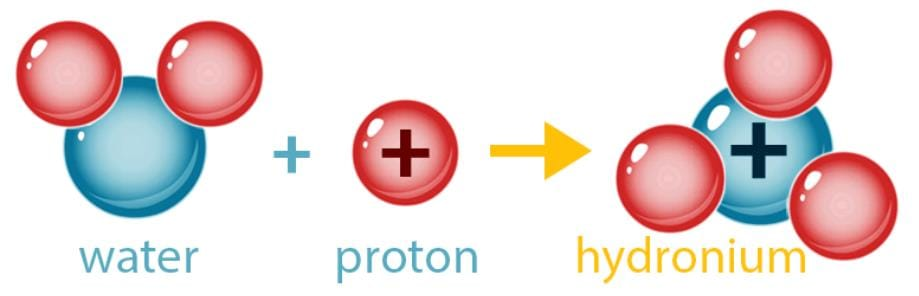• Acids are compounds that contain Hydrogen (Hydrochloric, HCl; Sulphuric, H2SO4; Nitric, HNO3). However, not all compounds that contain Hydrogen are acids (Water, H2O; Methane, CH4). Acids are usually compounds of non-metals with Hydrogen and sometimes Oxygen.
• Acids can be classified in various ways, depending on the factors mentioned below:
(i) Classification Based on the Strength of the acid.
(ii) Classification Based on the Basicity of the Acid.
(iii) Classification Based on the Concentration of the acid.
(iv) Classification Based on the presence of Oxygen.
• The strength of an acid depends on the concentration of the hydronium ions present in a solution. The greater the number of hydronium ions present, greater is the strength of acid. However, some acids do not dissociate to any appreciable extent in water such as carbonic acid. Therefore, these acids will have a low concentration of hydronium ions.
• Strong Acid: An acid, which dissociates completely or almost completely in water, is classified as a strong acid. It must be noted that in these acids all the hydrogen ions (H+) combine with water molecule and exist as hydronium ions (H3O+).
Examples of strong acids are: Hydrochloric acid, Sulphuric acid, Nitric acid etc.
• Weak Acid: An acid that dissociates only partially when dissolved in water, is classified as a weak acid. Most of the molecules remain in solution in molecular form itself in such acid.
Examples: Acetic acid, Formic acid, Carbonic acid, etc.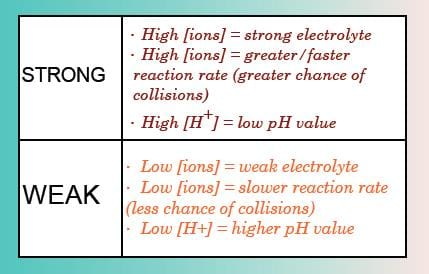Comparing strong and weak acids
• Acids are generally sour in taste. Special types of substances are used to test whether a substance is acidic or basic. These substances are known as indicators. The indicators change their color when added to a solution containing an acidic or a basic substance. Turmeric, litmus, china rose petals (Gudhal), etc., are some of the naturally occurring indicators.
• The most commonly used natural indicator is litmus. It is extracted from lichens. It has a mauve (purple) color in distilled water. When added to an acidic solution, it turns red and when added to a basic solution, it turns blue. It is available in the form of a solution, or in the form of strips of paper, known as litmus paper. Generally, it is available as red and blue litmus paper.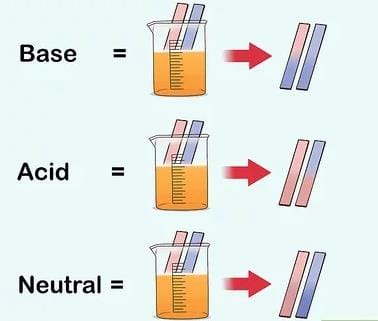Change in color of litmus paper
• The solutions which do not change the color of either red or blue litmus are known as neutral solutions. These substances are neither acidic nor basic.
• Acids are corrosive and can burn flesh and dissolve metal.

2. Bases and Alkalis

• A Base is a substance that gives OH ions when dissolved in water. Bases are usually metal hydroxides (MOH). Examples include Sodium Hydroxide, NaOH, Calcium Hydroxide, Ca(OH)2. The solution of a base in water is called an alkali.
• Bases and acids neutralize each other, therefore another way to define a base is a compound that reacts with an acid to give salt and water only. Like acids, alkalis can be strong or weak. The more hydroxide ions they produce, the stronger the alkali.
• The acidic property of an acid is due to the presence of hydrogen ions (H+) while that of a base or alkali, is due to the presence of hydroxyl (OH) ions in them. When an acid and base (alkali) combine, the positively charged hydrogen ion of the acid combines with the negatively charged hydroxyl ion of the base to form a molecule of water. Hence, the water molecule formed does not have any charge because the positive and negative charges of the hydrogen ions and hydroxyl ions get neutralized.
• The strength of a base depends on the concentration of the hydroxyl ions when it is dissolved in water.
(i) Strong Base: A base that dissociates completely or almost completely in water is classified as a strong base. The greater the number of hydroxyl ions the base produces, the stronger is the base.
Examples: Sodium hydroxide: NaOH, Potassium hydroxide: KOH, Calcium hydroxide: Ca(OH)2.
(ii) Weak Base: A base that dissociates in water only partially is known as a weak base.
Examples: Magnesium hydroxide: Mg(OH)2, Ammonium hydroxide: NH4OH.
• Bases are bitter to taste. They are soapy and slippery to touch. Strong alkalis like sodium hydroxide and potassium hydroxide are highly corrosive or caustic in nature. Sodium hydroxide and potassium hydroxide are commonly called caustic soda and caustic potash respectively. Organic tissues like skin, etc. get completely corroded by these two alkalis. However, the other alkalis are only mildly corrosive.

3. PH

• A scale for measuring hydrogen ion concentration in a solution, called pH scale has been developed. The p in pH stands for ‘potenz’ in German, meaning power. On the pH scale we can measure pH from 0 (very acidic) to 14 (very alkaline). pH should be thought of simply as a number that indicates the acidic or basic nature of a solution. Higher the hydronium ion concentration, lower is the pH value. The pH of a neutral solution is 7.
• Values less than 7 on the pH scale represent an acidic solution. As the pH value increases from 7 to 14, it represents an increase in OH ion concentration in the solution, that is, increase in the strength of alkali. Generally paper impregnated with the universal indicator is used for measuring pH.
• There are chemicals that change color at different pH values. These are called indicators. One of the most famous is Litmus. This substance turns red when the pH is less than 7 (acidic) and turns blue when the pH is greater than 7 (basic).

4. Salts

• A Salt results when an acid reacts with a base. Both are neutralized. The Hand OH- ions combine to form water. The non-metallic ions of the acid and the metal ions of the base form the salt.
• Important salts used in everyday life and industrial applications are Sodium chloride (NaCl), Sodium carbonate, (Na2CO3), Sodium Bicarbonate, (NaHCO3), Sodium Hydroxide (NaOH).
• The salt ions normally stay in the solution. The salt crystallizes out when the water is removed. Some salts are insoluble. They will precipitate out when the acid and base are added together.
• Salts of a strong acid and a strong base are neutral with pH value of 7. On the other hand, salts of a strong acid and weak base are acidic with pH values less than 7 and those of a strong base and weak acid are basic in nature, with pH values more than 7.

Atomic Structure

• An atom is the smallest particle of the element that can exist independently and retain all its chemical properties. Atoms are made up of fundamental particles: Electrons, Protons, and Neutrons.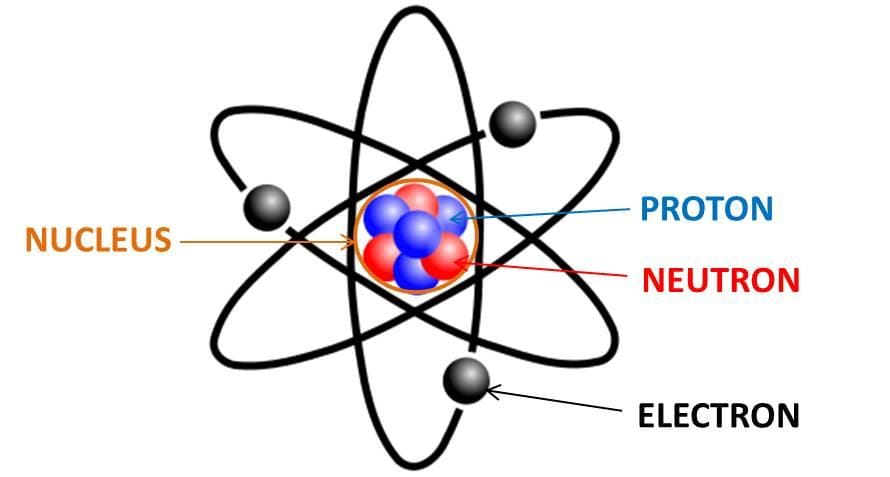An atom

Dalton’s Atomic Theory

John Dalton provided a simple theory of matter to provide theoretical justification to the laws of chemical combinations in 1805.

The basic postulates of the theory are:

• All substances are made up of tiny, indivisible particles called atoms.
• Atoms of the same element are identical in shape, size, mass and other properties.
• Each element is composed of its own kind of atoms. Atoms of different elements are different in all respects.
• Atom is the smallest unit that takes part in chemical combinations.
• Atoms combine with each other in simple whole-number ratios to form compound atoms called molecules.
• Atoms cannot be created, divided, or destroyed during any chemical or physical change.

Representation of an Atom by a Symbol

• Dalton was the first scientist to use the symbols for elements in a very specific sense. When he used a symbol for an element he also meant a  definite quantity of that element, that is, one atom of that element.
• A symbol signifies a shorthand representation of an atom of an element. The symbol of any element is based on the English name or Latin name (written in English alphabets) and many of the symbols are the first one or two letters of the element’s name in English. The first letter of a symbol is always written as a capital letter (uppercase) and the second letter as a small letter (lowercase).
Examples:
(i) Hydrogen- H
(ii) Aluminum- Al and not AL
(iii) Cobalt- Co and not CO.
Symbols of some elements are formed from the first letter of the name and a letter, appearing later in the name.
Examples:
(i) Chlorine - Cl
(ii) Zinc - Zn
• Other symbols have been taken from the names of elements in Latin, German, or Greek.
Examples:
(i) The symbol of iron is Fe from its Latin name Ferrum.
(ii) Sodium is Na from Natrium.
(iii) Potassium is K from Kalium.
Therefore, each element has a name and a unique chemical symbol.

Size of the Atom/ Elements

• Atoms are very small, they are smaller than anything that we can imagine or compare with. One hydrogen atom, the smallest atom known is approximately 5 x 10 mm in diameter. Atomic radius is measured in nanometres. 1 m = 109 nm.

Atomic Mass

• The mass of a particular atom is taken as a standard unit and the masses of other atoms are related to this standard. Hydrogen is the lightest element and is the smallest atom was chosen and assumed to have a mass of 1. An atom of hydrogen was assigned an atomic mass equal to one atomic mass unit (A.M.U).
• The number does not signify the mass of an atom in grams. It is just a pure number. The masses of atoms of other elements were compared to that of hydrogen, in order to find their atomic mass relative to it. If one atom of Sulphur weighs as much as 32 atoms of hydrogen, then the relative atomic mass of Sulphur is 32 A.M.U.
• This way of defining the mass of one atom of hydrogen has its difficulties. While the mass of one atom of hydrogen is considered as 1 atomic mass unit, hydrogen gas in its natural state has 3 isotopes of atomic mass 1, 2 and 3 respectively. Thus average mass works out to be 1.00 A.M.U rather than 1 A.M.U. This in turn complicates the atomic masses of all other elements.
• Later on, an atom of oxygen was preferred as standard by taking its mass as 16 units.
• However, in 1961 for a universally accepted atomic mass unit, carbon-12 isotope was chosen as the standard reference for measuring atomic masses.

One atomic mass unit is a mass unit equal to exactly one twelfth (1/12th) the mass of one atom of carbon-12

• The relative atomic masses of all elements have been found with respect to an atom of carbon-12. It is equal to 1.66 × 10-24g.

Molecule

• A molecule is in general, a group of two or more atoms that are chemically bonded together, that is, tightly held together by attractive forces.
• A molecule can be defined as the smallest particle of an element or a compound that is capable of independent existence and shows all the properties of that substance.
• Atoms of the same element or of different elements can join together to form molecules.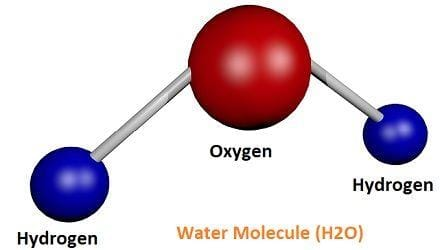• The molecules of an element are constituted by the same type of atoms. Molecules of many elements, such as Argon (Ar), helium (He) etc. are made up of only one atom of that element. But this is not the case with most of the nonmetals.
Example: A molecule of oxygen consists of two atoms of oxygen and hence it is known as a diatomic molecule, O2. If 3 atoms of oxygen unite into a molecule, instead of the usual 2, we get ozone. The number of atoms constituting a molecule is known as its atomicity.
• Atoms of different elements join together in definite proportions to form molecules of compounds. Compounds composed of metals and nonmetals contain charged species. The charged species are known as ions
• An ion is a charged particle and can be negatively or positively charged. A negatively charged ion is called an ‘anion’ and the positively charged ion, a ‘cation’. Take, for example, sodium chloride (NaCl). Its constituent particles are positively charged sodium ions (Na+) and negatively charged chloride ions (Cl). Ions may consist of a single charged atom or a group of atoms that have a net charge on them.
• A group of atoms carrying a charge is known as a polyatomic ion.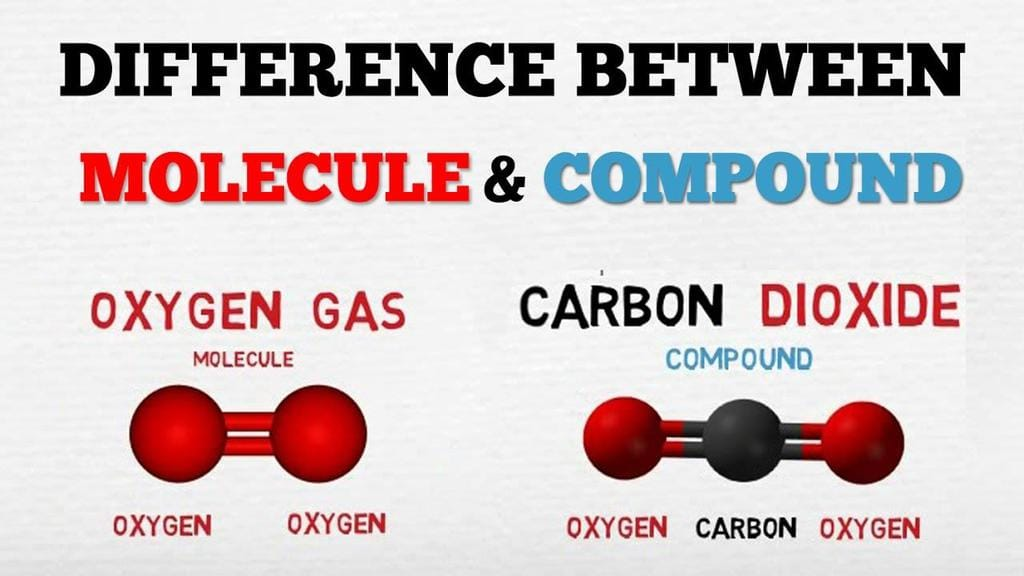Chemical Formulae

• The chemical formula of a compound is a symbolic representation of its composition. The chemical formulae of different compounds can be written easily.
• The combining power (or capacity) of an element is known as its valency. Valency can be used to find out how the atoms of an element will combine with the atom(s) of another element to form a chemical compound. The valency of the atom of an element can be thought of as hands or arms of that atom.
• The simplest compounds, which are made up of two different elements are called binary compounds. While writing the Chemical formula for compounds, we write the constituent elements and their valencies. Then we must crossover the valencies of the combining atoms.
• The formulae of ionic compounds are simply the whole number ratio of the positive to negative ions in the structure.

Molecular Mass

• The molecular mass of a substance is the sum of the atomic masses of all the atoms in a molecule of the substance. It is therefore the relative mass of a molecule expressed in atomic mass units (u).
• The formula unit mass of a substance is a sum of the atomic masses of all atoms in a formula unit of a compound. Formula unit mass is calculated in the same manner as we calculate the molecular mass. The only difference is that we use the word formula unit for those substances whose constituent particles are ions.
• Scientists use the relative atomic mass scale to compare the masses of different atoms of elements. Atoms of carbon-12 isotopes are assigned a relative atomic mass of 12 and the relative masses of all other atoms are obtained by comparison with the mass of a carbon-12 atom.

Mole Concept

• Since it is not possible to calculate the weight of particles individually, a collection of such particles called mole is taken for all practical purposes. It was discovered that the number of atoms present in 12g of carbon of 12C isotope is 6.023 × 1023 atoms. This is referred to as Avogadro number after the discoverer Avogadro.
• A mole of a gas is the amount of a substance containing 6.023 × 1023 particles. It is a basic unit of the amount or quantity of a substance. The substance may be atoms, molecules, ions or group of ions.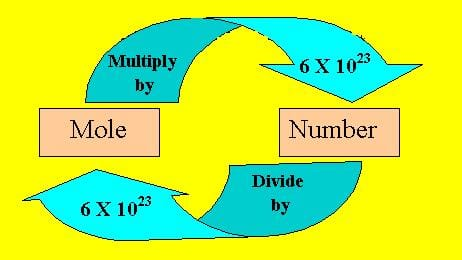• Mass of 1 mole of a substance is called its molar mass. One mole of any gas at STP will have a volume of 22.4 L. This is called the molar volume.
• Credit for the discovery of electron and proton goes to J.J. Thomson and E. Goldstein, respectively. J.J. Thomson proposed that electrons are embedded in a positive sphere.

Rutherford’s Model of the Atom

• Rutherford’s alpha-particle scattering experiment led to the discovery of the atomic nucleus. Rutherford’s model of the atom proposed that a very tiny nucleus is present inside the atom and electrons revolve around this nucleus. The stability of the atom could not be explained by this model.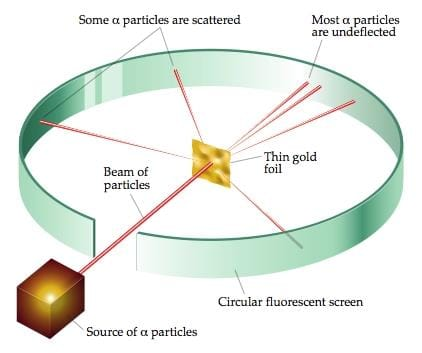Rutherford's Alpha-particle Scattering Experiment

Neils Bohr's Model

• Neils Bohr’s model of the atom was more successful. He proposed that electrons are distributed in different shells with discrete energy around the nucleus. If the atomic shells are complete, then the atom will be stable and less reactive.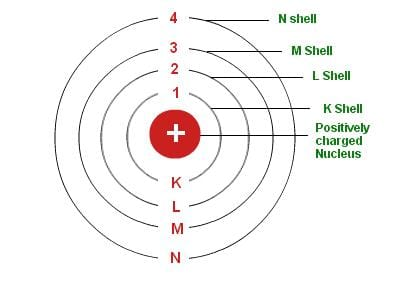Neil's Bohr's Model of the Atom

• J. Chadwick discovered presence of neutrons in the nucleus of an atom.
So, the three sub-atomic particles of an atom are:
(i) Electrons
(ii) Protons
(iii) Neutrons
Electrons are negatively charged, protons are positively charged and neutrons have no charges.
• The discovery of the electron, proton, and neutron was the starting point of new avenues of research in science, which gave physicists an insight into the structure and nature of the atoms of matter. An atom is made up of three elementary particles, namely electrons, protons, and neutrons.
• Electrons have a negative charge, protons have a positive charge and neutrons have no charge. Neutrons are neutral. Due to the presence of equal number of negative electrons and positive protons the atom as a whole is electrically neutral.
• Based on the above findings, one can say that the atom has two major divisions:
(i) The first is the centre of an atom, called its nucleus. The protons and neutrons are located in the small nucleus at the centre of the atom. Due to the presence of protons the nucleus is positively charged.
(ii) The second are electrons, which revolve around the nucleus in different shells (or orbits). Shells of an atom are designated as K, L, M, N,…. The space around the nucleus in which the electrons revolve determines the size of the atom.
• The maximum number of electrons present in a shell is given by the formula 2n2, where ‘n’ is the orbit number or energy level index, 1,2,3,…so on.
• Hence, the maximum number of electrons in different shells are as follows:
(i) First orbit or K-shell will be = 2.12 = 2
(ii) Second orbit or L-shell will be  = 2.22 = 8
(iii) Third orbit or M-shell will be = 2.32 = 18
(iv) Fourth orbit or N-shell will be = 2.42= 32, and so on.
• The maximum number of electrons that can be accommodated in the outermost orbit is 8. Electrons are not accommodated in a given shell unless the inner shells are filled. That is, the shells are filled in a step-wise manner.

Valency

• The electrons present in the outermost shell of an atom are known as the valence electrons. It is the decisive shell during a chemical reaction. The electrons of only this outermost shell are involved during chemical combinations, electrons are either given out from the outermost shell, or accepted into the outermost shell, or shared with the electrons in the outermost shell of another element.
• Elements having same number of valence electrons in their atoms possess similar chemical properties. The number of the valence shell in an atom determines its position in the Periodic Table i.e. the period to which the element belongs. Elements having 1, 2 or 3 electrons in the valence shell are metals. Exception is H and He. Elements having 4 to 7 electrons in their valence shell are non-metals.
• Valency is the combining capacity of an element. It is the number of electrons in an atom that actually take part in bond formation. For example, carbon atom with an atomic number 6 has 4 valence electrons.
• Calculation of Valency: The number of valence electrons is the valency of the element. The valency of an element can also be calculated by finding the number of electrons required to complete octet. If the outermost shell of an atom is completely filled, its valency = 0. The outermost shells of the noble gases helium, neon, argon, krypton etc. are completely filled. Hence their valency is zero. Such elements are very un-reactive and inert by nature.

Atomic Number

• The nuclei of atoms is made up of protons and neutrons. These two components of the nucleus are referred to as nucleons. The electrons occupy the space outside the nucleus.
• Since an atom is electrically neutral, the number of protons in the nucleus is exactly equal to the number of electrons. This number is the atomic number given by the symbol Z.

Mass Number

• The total number of protons and neutrons present in one atom of an element is known as its mass number.

Mass number = Number of protons + Number of neutrons

• Isotopes: Isotopes are atoms of the same element, which have different mass numbers. It is interesting to note that atoms of a given atomic number can have different numbers of neutrons. For example, take the case of hydrogen atom, it has three atomic species, namely protium (11 H), deuterium (21 H or D) and tritium (31 H or T). The atomic number of each one is 1, but the mass number is 1, 2 and 3, respectively.
• All isotopes of an element have the same number of valence electrons thus have identical chemical properties.
• The physical properties of the isotopes are different due to the difference in the number of neutrons in their nuclei. The densities, melting points and boiling points, etc., are slightly different.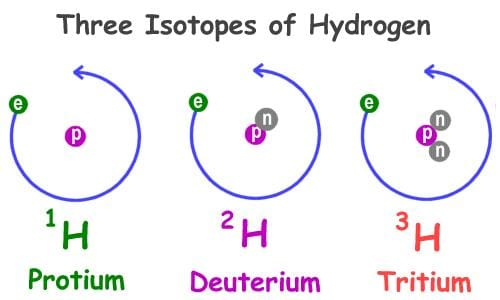• Isobars: Atoms of different elements with different atomic numbers, which have the same mass number, are known as isobars. These have different number of protons but equal sum of number of protons and neutrons.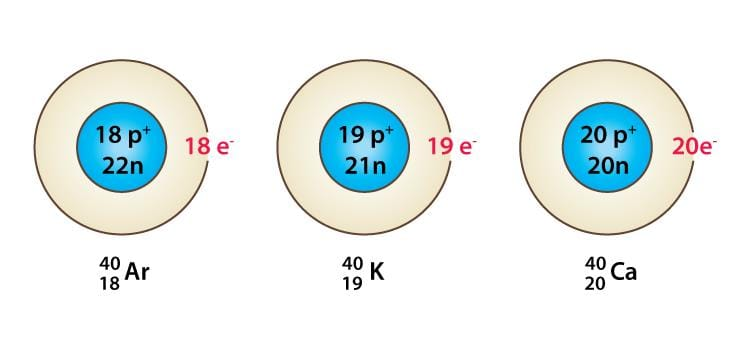Isobars
• Isotones: The atoms of different elements, which have the same number of neutrons but different atomic numbers, are called isotones.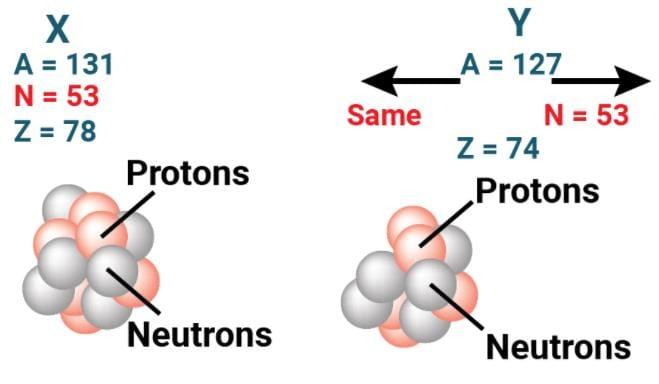Isotones

• Radioactivity is a nuclear phenomenon. It is the spontaneous emission of radiation from the nucleus.
• In 1899, the study of radioactivity was taken up by Ernest Rutherford. He placed a little radium at the bottom of a small lead box and subjected the rays that emerged from it to the action of a very strong magnetic field at right angles to their direction. He found that the rays separated into three distinct constituents.
• Rutherford called the three types of radiation alpha (α), beta (β), and gamma (γ) rays.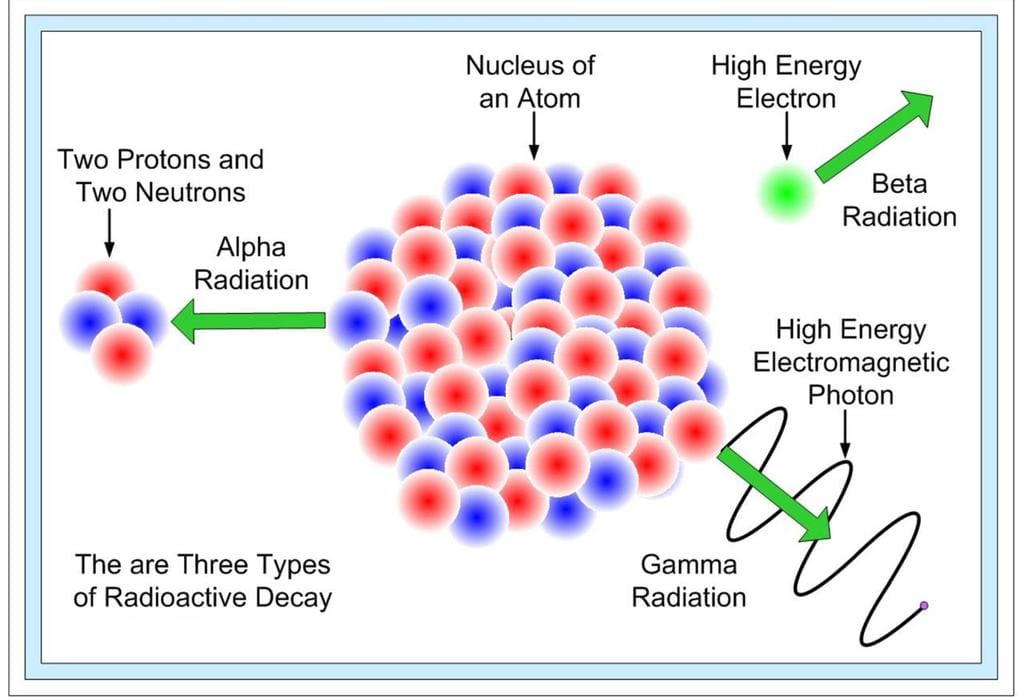Three types of Radioactive Decay
• The α-rays were deflected in a direction opposite to that of β-rays and α-rays carried a positive charge, β-rays carried a negative charge and those which passed undeviated were neutral or uncharged were γ-rays.
The document NCERT Summary: Summary of Chemistry- 1 | Science & Technology for UPSC CSE is a part of the UPSC Course Science & Technology for UPSC CSE.
All you need of UPSC at this link: UPSC

## Science & Technology for UPSC CSE

85 videos|355 docs|218 tests

## Science & Technology for UPSC CSE

85 videos|355 docs|218 tests

### How to Prepare for UPSC

Read our guide to prepare for UPSC which is created by Toppers & the best Teachers

Track your progress, build streaks, highlight & save important lessons and more!(Scan QR code)

,

,

,

,

,

,

,

,

,

,

,

,

,

,

,

,

,

,

,

,

,

;